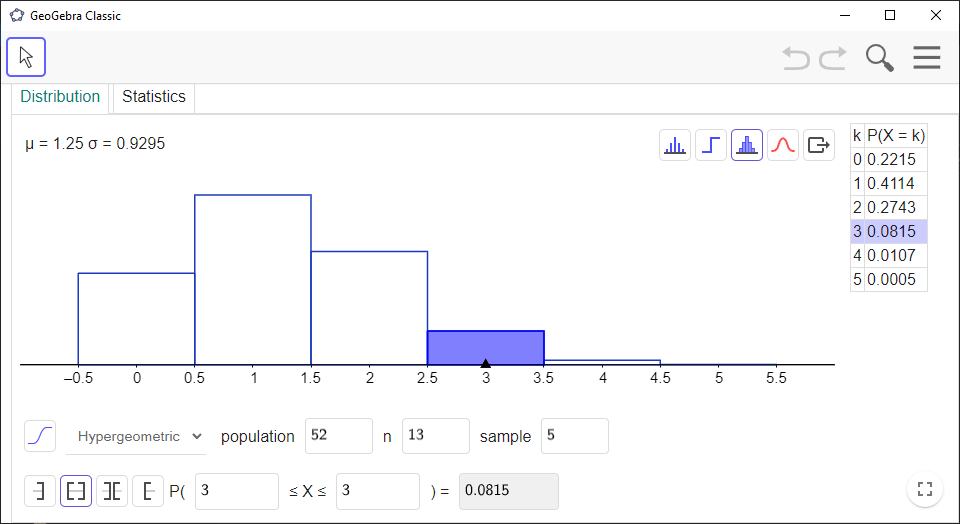# Hypergeometric Distribution Calculator in GeoGebra

Here’s how you can use the `Probability Calculator`in `GeoGebra` to compute probabilities from the hypergeometric distribution.

`GeoGebra` Instruction 1

1.
Open `Probability Calculator`under`View` in`Menu`.
2.
Make sure that you choose the `Distribution` tab at the top of the window.
3.
Select `Hypergeometric` from the drop-down list.

• `Population` is the total population.

• $n$ the number of elements in group $A$.

• `Sample` is the sample size, that is, the number of elements you draw.

4.
Choose `Left-sided`, `Interval`, `Two tailed`, or `Right-sided`depending on your problem.

• If you’re asked to compute the probability of getting exactly $k$ elements from $A$, you can find it in the table on the right-hand side.

• If you’re asked to compute the probability of getting at least $k$ elements from $A$, choose `Right-sided`and enter the value of $k$.

• If you’re asked to compute the probability of getting at most $k$ elements from $A$, choose `Left-sided`and enter the value of $k$.

• If you’re asked to compute the probability that the number of elements your draw from $A$ is between $N$ and $M$, including $N$ and $M$, choose `Interval`and enter the values of $N$ and $M$.

• If you’re asked to compute the probability that the number of elements your draw from $A$ is not between $N$ and $M$, choose `Two tailed` and enter the values of $N-1$ and $M+1$. Alternatively, you can compute the probability in the item above, and then subtract 1 by that probability.